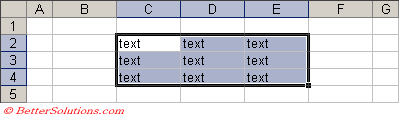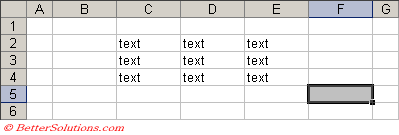# Finding Last Row Column

There are a number of ways to find the last row and/or column on a worksheet
0) Using Ctrl + Shift + End
1) Using the UsedRange property. (includes formatting)
2) Using SpecialCells - xlCellTypeLastCell. (includes formatting)
3) Using Cells.Find method.
4) Using End(XlDirection.xlUp)

### Using Ctrl + Shift + Enter

Extends the selection from the active cell to the last used cell on the worksheet.
Need to close and reopen ??

### Using the UsedRange

One of the most common ways to find the last cell on a worksheet is to use the UsedRange property.

`llastrow = ActiveSheet.UsedRange.Row + ActiveSheet.UsedRange.Rows.Count - 1 ilastcolumn = ActiveSheet.UsedRange.Column + ActiveSheet.UsedRange.Columns.Count - 1 `

When you have a "clean" worksheet the following code returns the correct last cell "E4".However this property does not just include cells that contain actual data but also any cells that have been formatted.
For example if we run the same code on the following worksheet the last cell is "F5".### Using SpecialCells - xlCellTypeLastCell

The same is true for the xlCellTypeLastCell argument which can be used with the SpecialCells property.

`llastrow = Range("A1").SpecialCells(xlCellType.xlCellTypeLastCell).Row ilastcolumn = Range("A1").SpecialCells(xlCellType.xlCellTypeLastCell).Column `

This also includes formatting in the range that is returned.
The xlLastCell constant is not documented because this constant was replaced in Excel 97 with the new xlCellType.xlCellTypeLastCell.
The following line of code does work but has only been included for backwards compatibility reasons.

`llastrow = Range("A1").SpecialCells(xlLastCell).Row `

The macro recorder will generate code with the xlLastCell constant which adds to the confusion.

### Using Cells.Find

Another popular approach which can be used to obtain the last "populated" row and column is to use the Cells.Find method.
This method searches backwards first by row and then by column from the top left cell.

`llastrow = ActiveSheet.Cells.Find(What:="*", _                                   After:=ActiveSheet.Range("A1"), _                                   SearchOrder:=xlSortOrder.xlByRows, _                                   SearchDirection:=xlSearchDirection.xlPrevious).Row llastrow = objRange.Find(What:=, After:=, SearchOrder:=xlByRows, SearchDirection).Row ilastcolumn = ActiveSheet.Cells.Find(What:="*", _                                      After:=ActiveSheet.Range("A1"), _                                      SearchOrder:=xlSortOrder.xlByColumns, _                                      SearchDirection:=xlSearchDirection.xlPrevious).Column llastcolumn = objRange.Find(What:=, After:=, SearchOrder:=xlByColumns, SearchDirection).Column `

### Using End(XlDirection.xlUp)

Use the following to obtain the address of the last non-empty cell in column "A".

`Dim llastrow As Long llastrow = Range(Range("A65536").End(xlDirection.xlUp).Address).Row `

### Checking each row or column at a time

The only way to accurately determine the next row or last row that contains data is to check every cell using ActiveCell.Offset(1,0).

### Last Column in a Range

`Set objRange = Range("B2:F20") icolumno = objRange(objRange.Rows.Count, objRange.Columns.Count).Column `